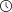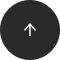- 9 minutes read

# How to Work with Python Date and Time Objects

At some point in your Python journey, you'll definitely need to work with dates, times, or both. Learn all the basics of date and time in Python with this short tutorial.

Need to calculate how long it's been since a given date? Working with date and time objects in Python? In this beginner's guide, we'll take a look at how to write programs with these two key data types in Python.

### Python Datetime Classes

Python has five classes for working with date and time objects:

• date – contains only date information (year, month, day).
• time – refers to time independent of the day (hour, minute, second, microsecond).
• datetime – combines date and time information.
• timedelta – represents the difference between two dates or times.
• tzinfo – an abstract class for manipulating time zone information.

These classes are supplied in the `datetime` module. So you'll need to start your work with date and time by importing all the required classes, like so:

```# Starting with the required imports
from datetime import datetime, date, time, timedelta
```

Now let's see what you can actually do with these classes.

#### Getting the Current Date and Time

First, it should come as no surprise that Python allows you to get the current date and time. You can also access the separate components of these objects.

To get the current date, you would use the `today()` method of the `date` class—that is, you'd write `date.today()`.

```# Getting current date
today = date.today()
days = ["Monday", "Tuesday", "Wednesday", "Thursday", "Friday", "Saturday", "Sunday"]
print ("Today's date is ", today)
print ("\n", "Year:", today.year,
"\n", "Month:", today.month,
"\n","Day:", today.day,
"\n","Weekday:", today.weekday(),
"\n","Weekday (name):", days[today.weekday()])```
```Today's date is  2019-02-22

Year: 2019
Month: 2
Day: 22
Weekday: 4
Weekday (name): Friday
```

This will return only today's date without the exact time information. However, the date has a set of components that you can access individually using the corresponding attributes of the date object. As you can see in the example above, it's possible to access the year, month, day, and even weekday.

Note that weekdays are provided as indices with 0 corresponding to Monday, 1 to Tuesday, 2 to Wednesday, and so on. So if you want to print the name of a weekday, you have two options:

• Create a list of strings that represent the names of the weekdays; access its individual elements using the output of the `date.weekday()` method as the index.
• Create a map of indices to their corresponding string names, and then use the index returned by `date.weekday()` as the key to the dictionary.

Neither approach is more efficient than the other, so use whichever one you prefer!

Now let's see how you can get the current time with Python, as well as access its individual components. Using the `now()` method of the datetime class, you'll get the full information about the current time, including the date. You can get only time information by passing in the output of `datetime.now()` to the `datetime.time()` method. Take a look at this example:

```# Getting current time
time = datetime.now()
print("It's", time, "now.")
print ("\n", "Time:", datetime.time(time),
"\n", "Hour:", time.hour,
"\n","Minute:", time.minute,
"\n","Second:", time.second,
"\n","Microsecond:", time.microsecond)
```
```It's 2019-02-23 13:10:12.387096 now.

Time: 13:10:12.387096
Hour: 13
Minute: 10
Second: 12
Microsecond: 387096
```

Similar to how we manipulated the `date` object, you can access the individual components of a time object using the corresponding attributes. Thus, you can separately access the current hour, minute, second, and microsecond.

### Creating Datetime Objects

Obviously, you can also create datetime objects in Python containing any plausible date and time from year 1 all the way up to year 9999. If you need an object that contains only date and no time information, simply pass in the year, month, and day to the `date()` function.

```# Creating date object
einstein_birthday = date(1879, 3, 14)
print ('Einstein\'s birthday is on {}.'.format(einstein_birthday))
```
```Einstein's birthday is on 1879-03-14.
```

```# Creating datetime object
einstein_birthtime = datetime(1879, 3, 14, 8, 34, 55, 2345)
print ('Einstein was born on {}.'.format(einstein_birthtime))
```
```Einstein was born on 1879-03-14 08:34:55.002345.
```

If we imagine for a second that the exact time of Einstein's birth were known, we could create a datetime object that contains not only the date but also the time of the event. To create such an object, we would pass in the necessary arguments to the `datetime()` function—that is, the year, month, day, hour, minute, second, and microsecond.

Note that year, month, and day are the only required arguments to the `datetime()` function; if you don't provide the other arguments, they'll be set to zero by default.

### Formatting Datetimes

Until now, the dates and times we've worked with were printed in the default format of "YYYY-MM-DD HH:MM:SS." However, Python gives you greater flexibility in how you format dates and times. Moreover, you'll need to master only one formatting method to get dates and times in any format you want: `strftime()`.

To change the format of your datetime object, you'll simply need to pass in the appropriate format to the `strftime()` method. The format is expressed using special directives like the following:

• %Y – year with century (e.g., 2019).
• %y – year without century (e.g., 19).
• %B – month as a full name (e.g., March).
• %b – month as an abbreviated name (e.g., Mar).
• %m – month as a zero-padded integer (e.g., 03).
• %d – day of the month as a zero-padded integer (e.g., 25).
• %A – weekday as a full name (e.g., Monday).
• %a – weekday as an abbreviated name (e.g., Mon).

Let's see how we can play with different formats:

```# Formatting a date object
einstein_birthday = datetime(1879, 3, 14)
print ('Einstein was born on {}.'.format(einstein_birthday))
print ('Einstein was born on {}.'.format(einstein_birthday.strftime('%b %d, %Y')))
print ('Einstein was born on {}.'.format(einstein_birthday.strftime('%a, %B %d, %Y')))
print ('Einstein was born on {}.'.format(einstein_birthday.strftime('%A, %B %d, %Y')))
print ('Einstein was born on {}.'.format(einstein_birthday.strftime('%d.%m.%Y')))
print ('Einstein was born on {}.'.format(einstein_birthday.strftime('%d/%m/%y')))
```
```Einstein was born on 1879-03-14 00:00:00.
Einstein was born on Mar 14, 1879.
Einstein was born on Fri, March 14, 1879.
Einstein was born on Friday, March 14, 1879.
Einstein was born on 14.03.1879.
Einstein was born on 14/03/79.
```

You can actually format time the same way, keeping in mind that:

• '%H' represents the hours in the 24-hour time format.
• '%I' represents hours in the 12-hour format.
• '%M' denotes the minutes.
• '%S' denotes the seconds.
• '%p' denotes whether the time is AM or PM.
```# Formatting a time object
meeting_time = datetime.time(datetime (2019, 3, 25, 20, 30))
print ('Meeting starts at {}.'.format(meeting_time))
print ('Meeting starts at {}.'.format(meeting_time.strftime('%H:%M')))
print ('Meeting starts at {}.'.format(meeting_time.strftime('%I:%M %p')))
```
```Meeting starts at 20:30:00.
Meeting starts at 20:30.
Meeting starts at 08:30 PM.
```

### Converting Strings into Datetime Objects

If dates or times in your dataset are represented as strings, you can actually easily convert them into datetime objects using the `strptime()` method. You simply need to specify the format in which your strings represent dates or times.

For example, let's suppose that you need to extract dates from a body of text where these dates are represented as "March 25, 2019." You'll just need to pass in this string and the corresponding format to the `strptime()` method. Take a look:

```# Converting strings to dates
date = datetime.strptime('March 25, 2019', '%B %d, %Y')
print(date)
```
```2019-03-25 00:00:00
```

Need more practice with converting strings into datetime objects in Python? Check out our course on working with strings in Python.

### Comparing Datetime Objects

Let's imagine that we know the exact birth time not only for Albert Einstein but also for Isaac Newton and Stephen Hawking. How do we compare date and time objects in Python?

Well, it's actually very straightforward! You can compare `date` and `datetime` objects the same way you'd compare numbers: with the greater-than (`>`) and less-than (`<`) operators.

```# Comparing times
einstein_birthtime = datetime(1879, 3, 14, 8, 34, 55, 2345)
newton_birthtime = datetime(1643, 1, 4, 15, 55, 4, 15432)
hawking_birthtime = datetime(1942, 1, 8, 22, 13, 43, 78432)
```
```einstein_birthtime > newton_birthtime
```
```True
```

```hawking_birthtime < einstein_birthtime
```
```False
```

### Getting Time Intervals

So we know that Einstein was born after Newton, but how much later? Let's find out!

```# Calculating the difference between two dates
delta = einstein_birthday - newton_birthday
print (delta)
print ('Einstein was born {} years later than Newton.'.format(round(delta.days/365)))```
```86266 days, 0:00:00
Einstein was born 236 years later than Newton.
```

As you can see from the example, you can calculate the difference between two date objects the same way you would with numbers. The result is expressed in days.

The delta that we calculated here is a `timedelta` object. It represents the difference between two dates or times. This difference can be expressed in days, seconds, microseconds, milliseconds, minutes, hours, and even weeks. Moreover, note that the result can be positive, negative, or zero.

Returning back to our physicists, let's find out how many days passed between Einstein's death and Hawking's birth:

```# Calculating the difference between two dates with a negative result
einstein_death = datetime(1955, 4, 18)
hawking_birthday = datetime(1942, 1, 8)
delta = hawking_birthday - einstein_death
print(delta)
```
```-4848 days, 0:00:00
```

We got a negative result because Einstein died when Hawking was already 13 years old.

### Creating timedelta Objects in Python

In the previous examples, `timedelta` objects resulted from our datetime arithmetic operations, but we can also create these objects explicitly and use them for defining new date and time objects. For example, given a specific date, we can calculate a new date by adding or subtracting a particular number of days, hours, weeks, etc.

```# Calculating the date 100 days before Einstein's birth
einstein_100days_before = einstein_birthday + timedelta(days=-100)
print ('Einstein was born 100 days after {}.'.format(einstein_100days_before))
```
```Einstein was born 100 days after 1878-12-04 00:00:00.
```

```# Calculating the date 4 weeks after Einstein's birth
einstein_4weeks_after = einstein_birthday + timedelta(weeks=4)
print ('Einstein was 4 weeks old on {}.'.format(einstein_4weeks_after))
```
```Einstein was 4 weeks old on 1879-04-11 00:00:00.
```

As you can observe from the examples, when we pass in a negative number as an argument to `timedelta()`, the given duration is subtracted from the original date (e.g., minus 100 days). On the other hand, when we pass in a positive number, we add this duration to our date object (e.g., plus 4 weeks).

### Python Date and Time—Learned!

Now you know how to create datetime objects from scratch or from strings, how to get the current date and time, how to compare two dates, and how to format dates and times. You know all the basics, so your next step is to practice! And for that, be sure to check out our Python Basics Part 3 course; it has tons of interactive exercises and some quizzes related to date and time manipulation in Python.

You may also find it useful to check out Python Basics Part 1 and Python Basics Part 2 to refresh your knowledge of conditional statements, loops, lists, dictionaries, and other essential Python programming concepts.

If you want to observe considerable progress in your career, make sure to invest some time and effort in professional development and continuing education. You never know which of these newly acquired skills will play a crucial role in your next promotion—but they all definitely will!